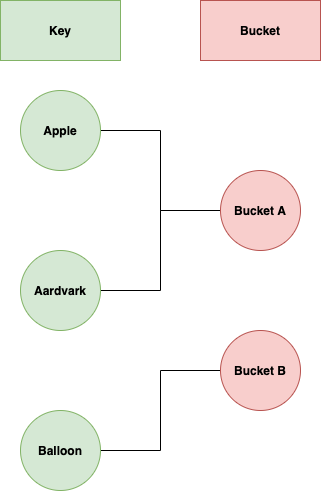# Argument

Hash Tables organise data using hash functions to support quick insertion and search. There are two types of hash table: hash set and hash map.

• Hash Set: A set of unique values.
• Hash Map: An implementation of map, which stores a set of unique keys, mapping to values.

Hash Tables map keys to buckets. We use a hash function on a key to generate a hash, then use this hash to map to a bucket.Example of mapping keys to buckets using a hash function which takes the first letter

In the above our hash function takes the first letter of the key. To search for a key we hash it, find the bucket, then search inside for the value.

## Designing a Hash Function

Making an effective hash function is one of our key challenges. It should be as uniform as possible to ensure a good spread of keys to buckets. It will depend on:

1. The range of key values. For example, if all of our previous keys began with different letters, we would have a good hash function.
2. The number of buckets. The more buckets we have available, the more unique hash values we can have.
3. The capacity of the buckets. Previously we mapped two keys to the same bucket. If our buckets have a high capacity we can have more keys pointing to the same one.

As we saw with ‘Apple’ and ‘Aardvark’, collisions are inevitable. How we resolve this depends on our collision resolution algorithm. This determines:

1. How values are organised in a bucket.
2. Behaviour if too many values are added to the same bucket.
3. How to search a bucket for a value.

We may use an array or height-balanced binary tree to store values in the bucket.

## Complexities

From the above we have:

• Insertion complexity `O(1)`
• Search complexity (best case `O(1)`, single entry in a bucket, worst case `O(log(n))` , searching a height-balanced binary tree).

## Implementations and Problems

Below is an implementation of a Hash Set. It assumes a range of integer keys between `0`and `1000000`.

We can then extend this idea to Hash Map, where all of the values are also integers.

Now we have some implementation ideas, we need to understand what kind of problems Hash Functions, Hash Sets and Hash Maps are used for.

In general, if a problem:

1. Counts the number of occurrences of certain events.
2. Searches for something in common.
3. Requires a check for uniqueness.

Then a Hash Map or Hash Set is a good place to start. Let’s dive into some LeetCode problems to have a closer look.

Given an array of strings `strs`, group the anagrams together. You can return the answer in any order.

We can solve this by looping through the array of strings and ordering the characters. This is our key. Each time we find another matching key we add to the value, which is our list of anagrams.

Given two integer arrays `nums1` and `nums2`, return an array of their intersection. Each element in the result must appear as many times as it shows in both arrays

We can solve this by looping through the first array, creating a map where the key is the integer, and the value is the number of occurrences. We can do the same for the second array.

We then look for the intersection of keys (the shared elements), and take the minimum of the values (the number of shared repetitions of that element).

Determine if a `9 x 9` Sudoku board is valid. Only the filled cells need to be validated according to the following rules:

Each row must contain the digits `1-9` without repetition.

Each column must contain the digits `1-9` without repetition.

Each of the nine `3 x 3` sub-boxes of the grid must contain the digits `1-9` without repetition.

The clue that we need a hash set for this solution is the fact that we require no repetition. This suggests we need to be able to look up values to check if we have seen them before. This can be done in `O(1)` time by using a Hash Set. The rest of the exercise is then unfurling the rows, columns and boxes into arrays and running them through our checker.

Given the `root` of a binary tree, return all duplicate subtrees.

Again we see a need for uniqueness, suggesting a hash function. We can recurse down a tree, generating a hash using the values at each of the nodes. This will be our key. During our recursion we add each subtree’s hash as a key, incrementing a counter (the value) as we encounter more instances.

# Conclusion

In conclusion, we have covered the definition of Hash Tables, Hash Sets and Hash Maps. We have also looked at a brief implementation, and outlined the sort of problems we expect to apply these concepts to.

--

--